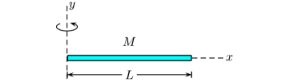# Problem: A nonuniform rod lies along the x-axis with its left end at x = y = 0 . It has a total mass M , length L and a mass distribution of dM / dx = B (L2 − x2), where B is a constant to be found in your analysis. What is the rotational inertia of the rod about the y-axis, pivoting about a vertical axis through its left end? 1. 1/2 ML2 2. ML2 3. 1/4 ML2 4. 2/3 ML2 5. 3/5 ML2 6. 1/7 ML2 7. 1/3 ML2 8. 1/6 ML2 9. 1/5 ML2 10. 3/4 ML2

98% (18 ratings)
###### Problem Details

A nonuniform rod lies along the x-axis with its left end at x = y = 0 . It has a total mass M , length L and a mass distribution of dM / dx = B (L2 − x2), where B is a constant to be found in your analysis. What is the rotational inertia of the rod about the y-axis, pivoting about a vertical axis through its left end?

1. 1/2 ML2

2. ML2

3. 1/4 ML2

4. 2/3 ML2

5. 3/5 ML2

6. 1/7 ML2

7. 1/3 ML2

8. 1/6 ML2

9. 1/5 ML2

10. 3/4 ML2